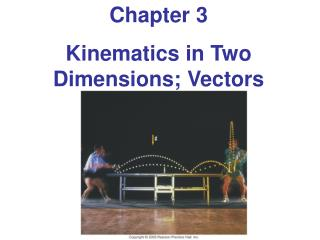Download PresentationChapter 3 Kinematics in Two Dimensions; Vectors

Chapter 3 Kinematics in Two Dimensions; Vectors - PowerPoint PPT Presentation

Chapter 3 Kinematics in Two Dimensions; Vectors. Units of Chapter 3. Projectile Motion Solving Problems Involving Projectile Motion Projectile Motion Is Parabolic. 3-4 Adding Vectors by Components.I am the owner, or an agent authorized to act on behalf of the owner, of the copyrighted work described.
Download PresentationChapter 3 Kinematics in Two Dimensions; Vectors

Download Policy: Content on the Website is provided to you AS IS for your information and personal use and may not be sold / licensed / shared on other websites without getting consent from its author.While downloading, if for some reason you are not able to download a presentation, the publisher may have deleted the file from their server.

- - - - - - - - - - - - - - - - - - - - - - - - - - E N D - - - - - - - - - - - - - - - - - - - - - - - - - -
Presentation Transcript
1. Chapter 3 Kinematics in Two Dimensions; Vectors

2. Units of Chapter 3 • Projectile Motion • Solving Problems Involving Projectile Motion • Projectile Motion Is Parabolic

3. 3-4 Adding Vectors by Components Any vector can be expressed as the sum of two other vectors, which are called its components. Usually the other vectors are chosen so that they are perpendicular to each other.

4. 3-4 Adding Vectors by Components If the components are perpendicular, they can be found using trigonometric functions.

5. 3-4 Adding Vectors by Components • Adding vectors: • Draw a diagram; add the vectors graphically. • Choosex and y axes. • Resolve each vector into x and ycomponents. • Calculate each component using sines and cosines. • Add the components in each direction. • To find the length and direction of the vector, use:

6. 3-5 Projectile Motion A projectile is an object moving in two dimensions under the influence of Earth's gravity; its path is a parabola.

7. 3-5 Projectile Motion It can be understood by analyzing the horizontal and vertical motions separately.

8. 3-5 Projectile Motion The speed in the x-direction is constant; in the y-direction the object moves with constant accelerationg. This photograph shows two balls that start to fall at the same time. The one on the right has an initial speed in the x-direction. It can be seen that vertical positions of the two balls are identical at identical times, while the horizontal position of the yellow ball increases linearly.

9. 3-5 Projectile Motion If an object is launched at an initial angle of θ0 with the horizontal, the analysis is similar except that the initial velocity has a vertical component.

10. 3-6 Solving Problems Involving Projectile Motion Projectile motion is motion with constant acceleration in two dimensions, where the acceleration is g and is down.

11. 3-6 Solving Problems Involving Projectile Motion • Read the problem carefully, and choose the object(s) you are going to analyze. • Draw a diagram. • Choose an origin and a coordinate system. • Decide on the time interval; this is the same in both directions, and includes only the time the object is moving with constant acceleration g. • Examine the x and y motions separately.

12. 3-6 Solving Problems Involving Projectile Motion 6. List known and unknown quantities. Remember that vx never changes, and that vy = 0 at the highest point. 7. Plan how you will proceed. Use the appropriate equations; you may have to combine some of them.

13. 3-7 Projectile Motion Is Parabolic In order to demonstrate that projectile motion is parabolic, we need to write y as a function of x. When we do, we find that it has the form: This is indeed the equation for a parabola.